﻿ 一种特殊的非均匀水声阵列稀疏重构方法
 舰船科学技术2018, Vol. 40Issue (3): 132-136PDF

1. 江苏科技大学 电子信息学院，江苏 镇江 212003;
2. 水声对抗技术重点实验室，北京 100094

A special method for non-uniform acoustic array with sparse reconstruction
YAN Yu-xia1, WANG Biao2
1. School of Electronic and Information, Jiangsu University of Science and Technology, Zhenjiang 212003, China;
2. Key Laboratory of Underwater Acoustic Warfare Technology, Beijing 100094, China
Abstract: For improving the utilization ratio of the array elements and the precision of underwater targets, a special sparse reconstruction approach for NULA are proposed. This NULA which composed of two linear uniform sub-arrays is used as the receiving array of signal. Flow pattern array of NULA which have divided angle is the observation matrix. Using the observation matrix for projection measurement of the signal to obtain observed value. The original signal is reconstructed from the observed value then getting the orientation information. The technology of Non-uniform acoustic array can use fewer elements to identify more underwater targets with the same resolution condition. So it greatly reducing the complexity of traditional underwater acoustic array. It also improve the direction-finding accuracy of underwater acoustic array in low SNR and low prior knowledge conditions.
Key words: non-uniform linear array (NULA)     compressed sensing     sparse reconstruction     estimation of the direction of arrival
0 引　言

1 阵列信号模型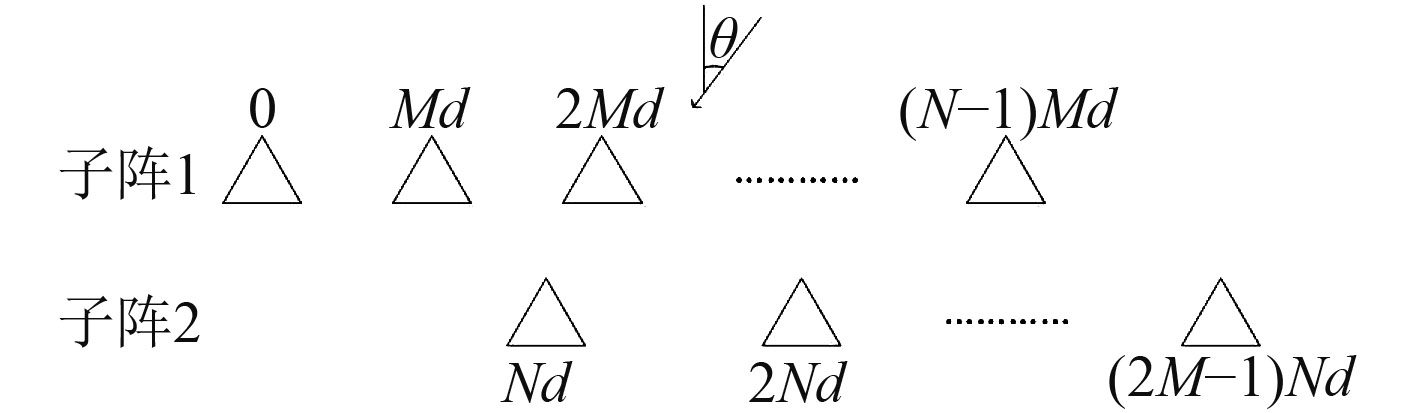图 1 均匀线性子阵列 Fig. 1 Uniform linear sub-array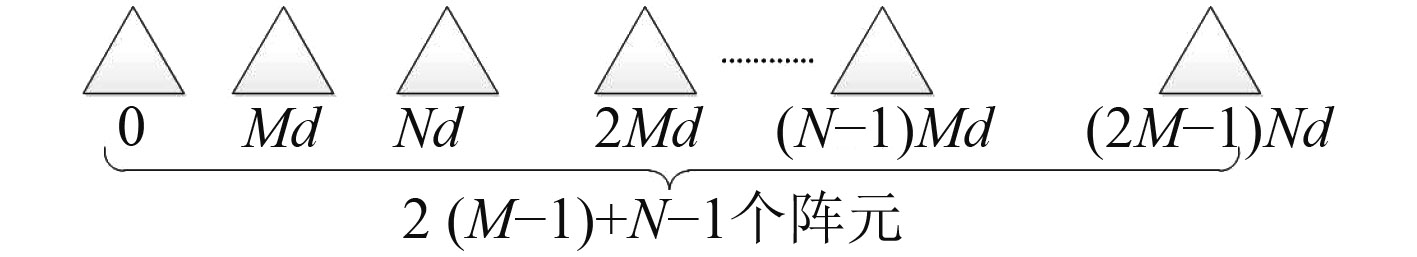图 2 非均匀线阵 Fig. 2 Non-uniform linear array

 $x\left( t \right) = \sum\limits_{k = 1}^K {a\left( {{\theta _k}} \right)} {s_k}\left( t \right) + n\left( t \right) = {{A}}\left( \theta \right)s\left( t \right) + n\left( t \right)\text{。}$ (1)

2 稀疏重构模型

 $y\left( t \right) = \varPhi x\left( t \right) = \varPhi {{A}}\left( \theta \right)s\left( t \right) + \varPhi n\left( t \right)\text{，}$ (2)

 $Y = \varPhi X = \varPhi {{A}}S + N,$ (3)

 $\min {\left\| S \right\|_{{l_1}}},\;{ s.t}\;{\left\| {Y - \Phi AS} \right\|_{{l_2}}} \leqslant \varepsilon \text{。}$ (4)
3 特殊的非均匀水声阵列稀疏重构方法 3.1 特殊的非均匀阵列分析

 $\begin{split}& {y_r} = E[{x_p}\left( t \right){x_q}^H\left( t \right)] = \sum\limits_{k = 1}^K {\sigma _k^2} {a_p}\left( {{\theta _k}} \right){a_p}^H\left( {{\theta _k}} \right) +\\& \sigma _n^2I = {{A}}P{{{A}}^{ H}} + \sigma _n^2I\text{。}\end{split}$ (5)

 $\widehat {{y_r}} = \frac{1}{L}\sum\limits_{l = 1}^L {{x_p}} \left( t \right){x_q}\left( t \right)\text{。}$ (6)
3.2 特殊的非均匀阵列稀疏重构方法

 $z = vec\left( {\widehat y} \right) = \widetilde A{ b} + \sigma _n^2\widetilde i\text{。}$ (7)

 $\widehat b = \arg \min {\left\| b \right\|_0}\text{，}\;\;{{subject}}\;{{to}}\;\;{\left\| {z - \widetilde Ab - \sigma _n^2\widetilde i} \right\|_2} < \varepsilon \text{。}$ (8)

 $\widehat r = \arg \;\mathop {\min }\limits_r {\left\| b \right\|_0}\text{，}\;\;{ subject \;to}\;\;{\left\| {z - Br} \right\|_2} < \varepsilon \text{。}$ (9)

 $B\left( \theta \right) = \left[ {a\left( {{\theta _1}} \right),a\left( {{\theta _2}} \right),\; \cdots ,a\left( {{\theta _Q}} \right)} \right]\text{。}$ (10)

4 仿真结果与分析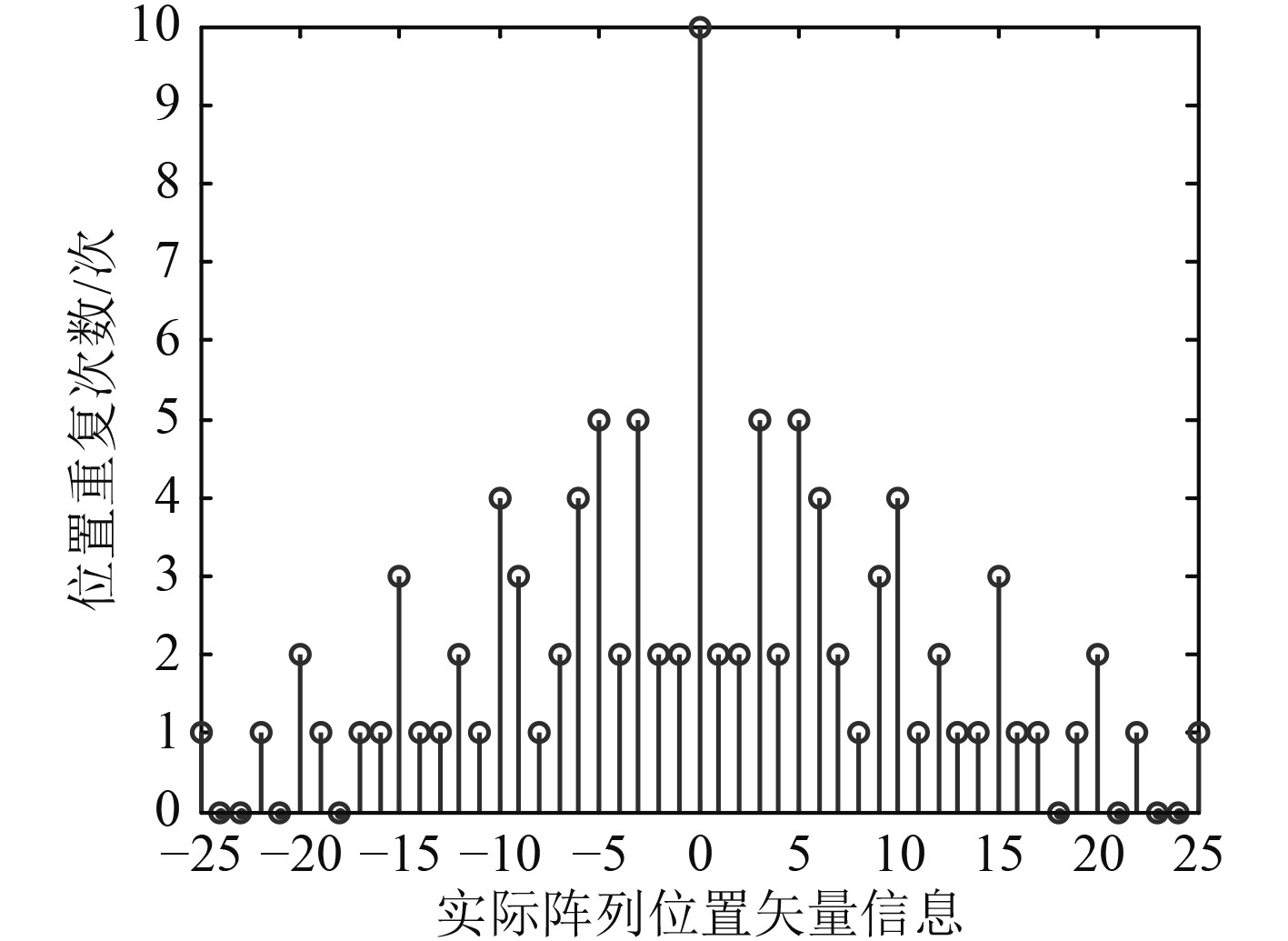图 3 实际阵列位置矢量信息 Fig. 3 Vector information of actual array position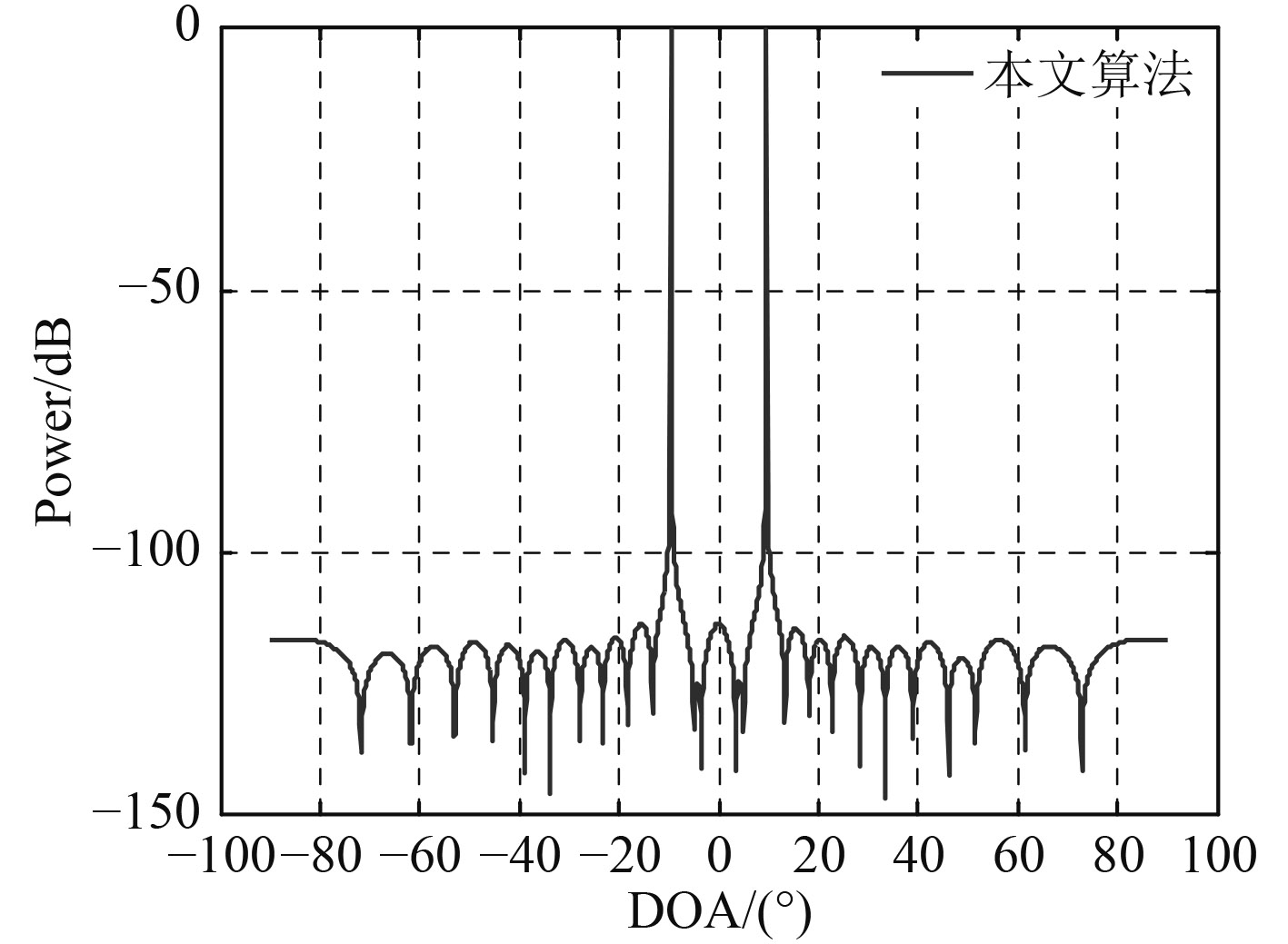图 4 本文方法实现DOA估计 Fig. 4 Proposed method for DOA estimation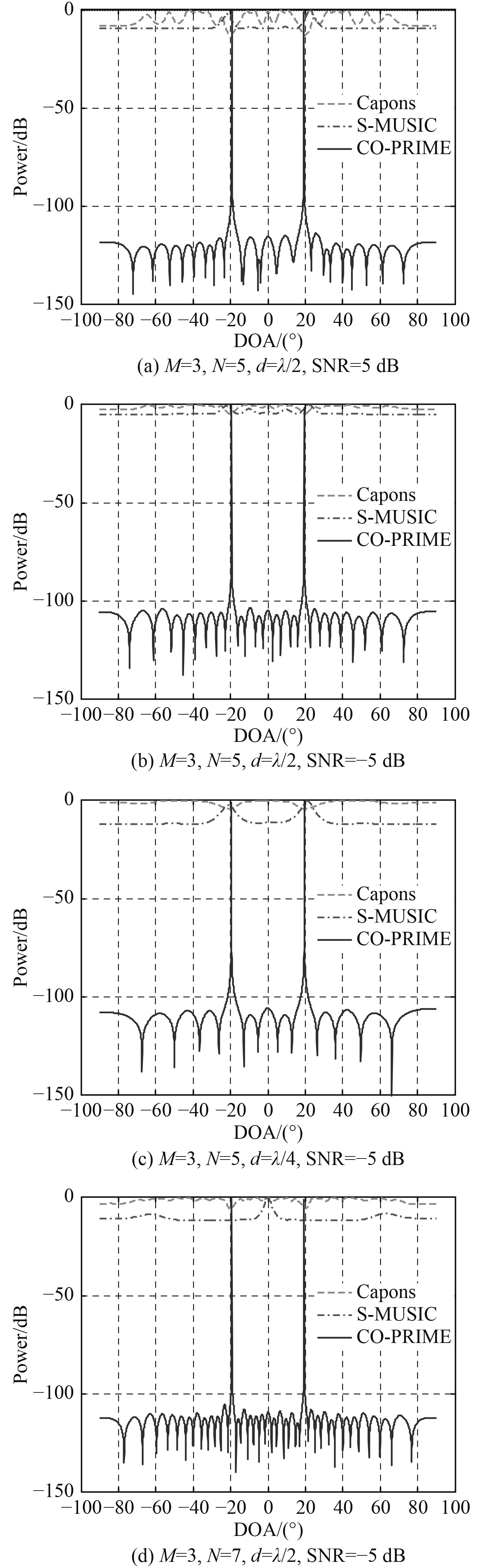图 5 不同参数条件下各种方法实现信号DOA估计比较结果 Fig. 5 The DOA estimation results of different method with different parameters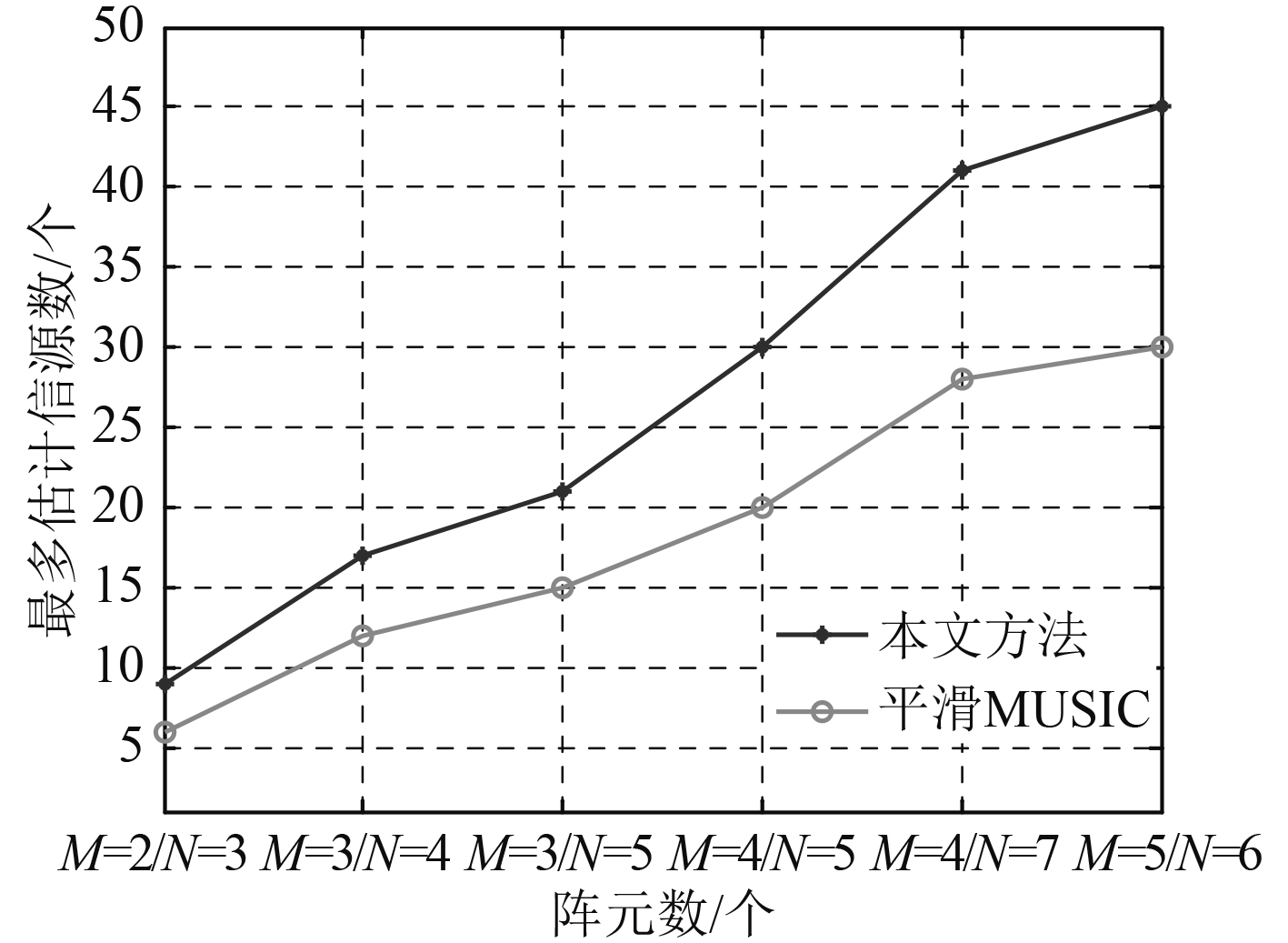图 6 可估计的最多信源数比较 Fig. 6 The estimated maximum number of source comparison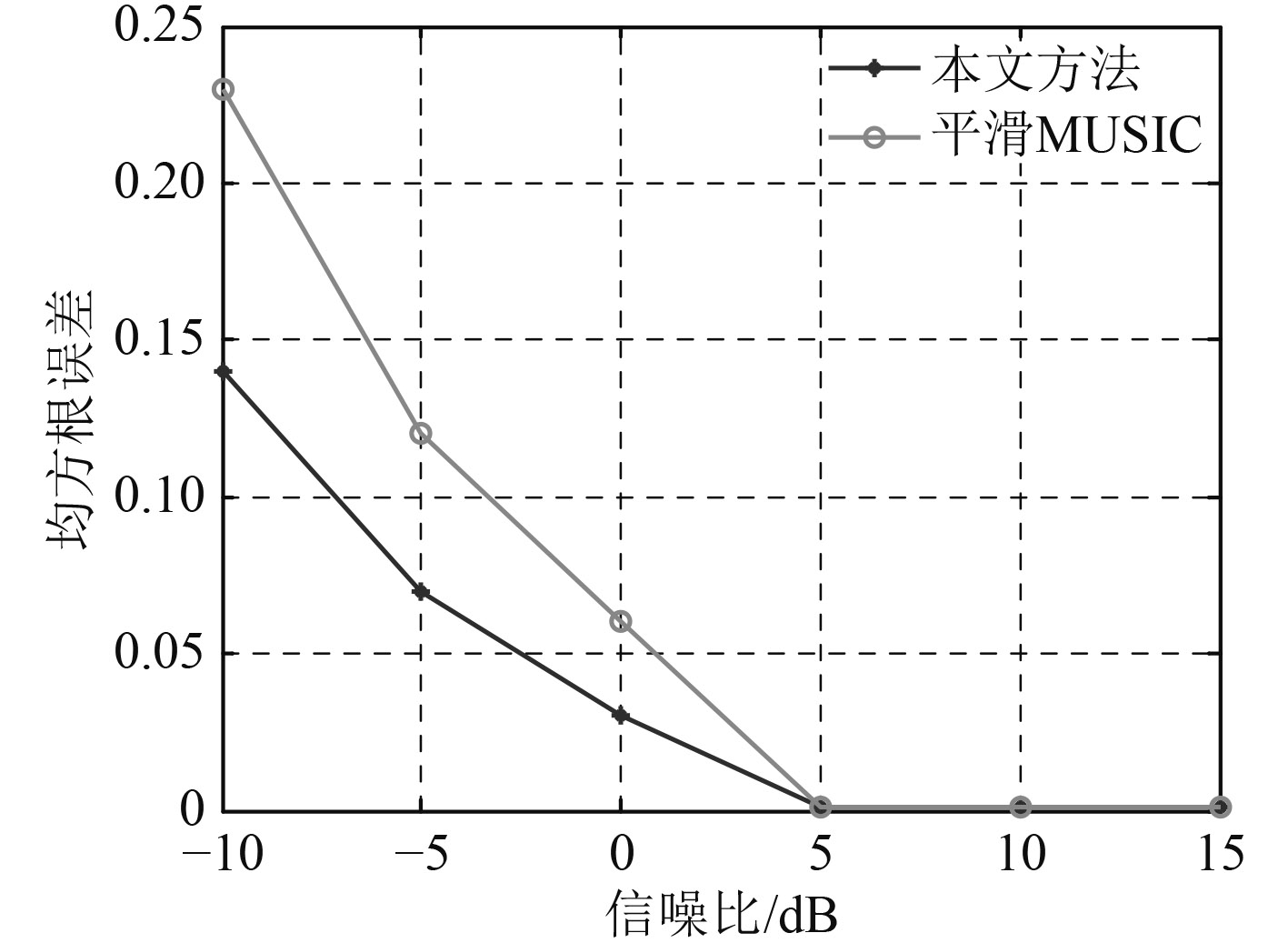图 7 两种估计方法均方根误差比较 Fig. 7 The RMSE comparison of the two estimation methods
5 结　语

  王永良, 陈辉, 彭应宁, 等. 空间谱估计理论与算法[M]. 北京: 清华大学出版社, 2004: 82–138. WANG Yong-liang, CHEN Hui, PENG Ying-ning, et al. Spatial spectrum estimation theory and algorithms[M]. Beijing: Academic Building, Tsinghua University Press, 2004: 82–138.  张峰. 水下目标DOA估计方法研究[D]. 哈尔滨: 哈尔滨工程大学. 2013. 3: 11–12. ZHANG Feng. Research on DOA estimation method of underwater target[D]. Harbin: Harbin Engineering University. 2013. 3: 11–12.  XIANG Yang, HUANG Jiao-guo, SHI Wen-tao, et al. High resolution direction-of-arrival estimation based on beam –spectra envelope[C]// Signal Processing, Communication and Computing(ICSPCC), 2013 IEEE International Conference on, Kunming, 2013: 1–2.  YAN Feng-gang, JIN Ming, QIAO Xiao-lin. Low-complexity DOA estimation based on compressed MUSIC and its performance analysis[J]. IEEE Transactions on Signal Processing, 2013. 4, 61(8): 1915–1916.  PASCAL V, XAVIER M, PHILIPPE L. Performance analysis of an improved MUSIC DoA estimator[J]. IEEE Transactions on Signal Processing, 2015. 8, 63(23): 6407–6408.  OLLIER V, EL KORSO M. N, BOYER R, et al. Joint ML calibration and DOA estimation with separated arrays[C]// 2016 IEEE International Conference on Acoustics, Speech and Signal Processing(ICASSP), Shanghai, 2016: 2996–2998.  田达, 张洪, 陈天麒. 一种新的时频极大似然DOA估计方法[J]. 电子与信息学报, 2005. 2, 27(2): 2–3. TIAN Da, ZHANG Hong, CHEN Tian-lin. DOA estimation based on time-frequency maximum likelihood[J]. Journal of Electronics & Information Technology, 2005. 2, 27(2): 2–3. http://www.cqvip.com/QK/91130A/200502/15001594.html  PIYA P, VAIDYANATHAN P P. Non uniform linear arrays for improved identifiability in cumulant based DOA estimation[C]// 2011 Conference Record of the Forty Fifth Asilomar Conference on Signals, Systems and Computers (ASTLOMAR), 2011: 609–610.  陈柱学, 吴建新. 非均匀线阵的快速求根MUSIC方法[J]. 雷达科学与技术, 2014. 4, 12(2): 167–169. CHEN Zhu-xue, WU Jian-xin. Fast root MUSIC for non-uniform linear array[J]. Redar Science and Technology, 2014. 4, 12(2): 167–169. http://d.wanfangdata.com.cn/Periodical_ldkxyjs201402010.aspx  王彪, 李超, 戴跃伟. 基于空域压缩采样的水声目标DOA估计方法[J]. 兵工学报, 2013. 11, 34(11): 1480–1481. WANG Biao, LI Chao, DAI Yue-wei. DOA estimation method based on spatial compressive sampling for underwater acoustic target[J]. Acta Armamentarii, 2013. 11, 34(11): 1480–1481. http://manu48.magtech.com.cn/Jwk_bgxb/CN/abstract/abstract234.shtml  GUO Xin, SUN Hong-bo. Cpmpressive sensing for target DOA estimation in radar[C]// 2014 International Radar conference, Lille, 2014: 1–2.  MASATO G, YOSHIKI T, ATSUO O. Fast implementation of sparse reconstruction for CS-based DoA estimation[C]// Radar Conference(EuRAD), Paris, 2015: 165–166.  MALIOUTOV D, CETIN M, WILLSKY A. A sparse signal reconstruction perspective for source localization with sensor arrays[J]. IEEE Transactions on Signal Processing, 2005, 53(8): 3010–3022.  KULAIB A R, SHUBAIR R M, QUTAYRI M Al, et al. Accurate and robust DOA estimation using uniform circular displaced antenna array[C]// 2015 IEEE International Symposium on Antennas and Propagation & USNC/URSI National Radio Science Meeting, 2015: 1552.  Gu Jian-feng, ZHU Wei-ping, SWAMY M N S. Fast and accurate 2-D DOA estimation via sparse L-shaped array[C]// 2014 IEEE International Symposium on Circuits and Systems(ISCAS), 2014, 10: 1292–1293.  PIYA P, VAIDYANATHAN P P. Non uniform linear arrays for improved identifiability in cumulant based DOA Estimation[C]// 2011 Conference Record of the Forty Fifth Asilomar Conference on Signals, Systems and Computers (ASILOMAR). 2011, 10(1): 608–609.  杨桂芹, 房琪, 胡滢. 基于非均匀阵列天线的MUSIC算法性能分析[J]. 兰州交通大学学报, 2012, 31(6): 88–89. YANG Gui-qin, FANG Qi, HU Ying. Performance analysis of MUSIC algorithm based on non-uniform array antenna[J]. Journal of Lanzhou Jiaotong University, 2012, 31(6): 88–89. http://www.cnki.com.cn/Article/CJFDTOTAL-LZTX201206021.htm  ZHANG Yi-min, QIN Si, MONEESS G A. DOA estimation exploiting coprime arrays with sparse sensor spacing[C]// IEEE International Conference on Acoustic, Speech and Signal Processing (ICASSP), Florence, 2014: 2267–2268.  HU Nan, YE Zhou-fu, XU Xu, et al. DOA estimation for sparse array via sparse signal reconstruction[J]. IEEE Transactions on Aerospace and Electronic Systems, 2013, 49(2): 761–773.  QIN Si, ZHANG Yi-min, MOENESS G A. Generalized coprime array configurations for direction-of-arrival esitimation[J]. IEEE Transactions on Signal Processing, 2015, 63(6): 1377–1378.